# 模糊聚类分析基本知识

（1）自反性

（2）对称性

（３）传递性

## 2. 模糊等价关系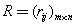只满足自反性和对称性，则称为相似关系。

# 系统聚类法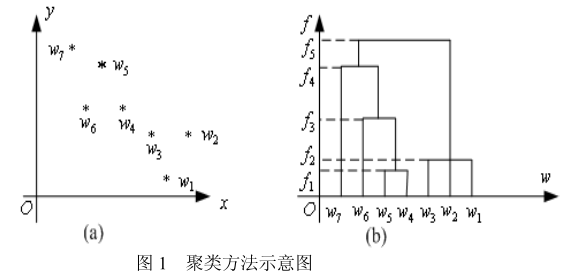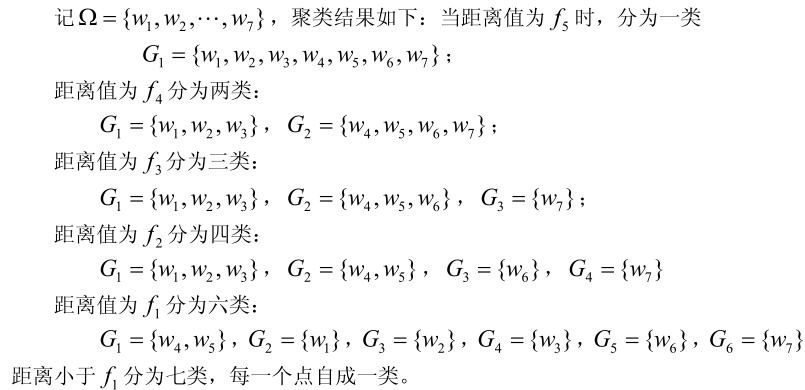1）计算 n 个样本点两两之间的距离{dij} ，记为矩阵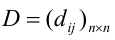2）首先构造 n 个类，每一个类中只包含一个样本点，每一类的平台高度均为零；
3）合并距离最近的两类为新类，并且以这两类间的距离值作为聚类图中的平台高

4）计算新类与当前各类的距离，若类的个数已经等于 1，转入步骤 5），否则，回到步骤 3）；
5）画聚类图；
6）决定类的个数和类。

# 示例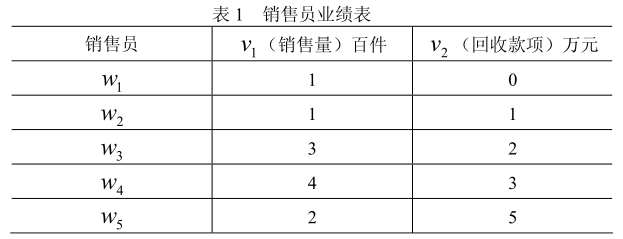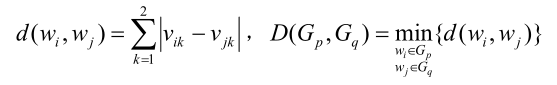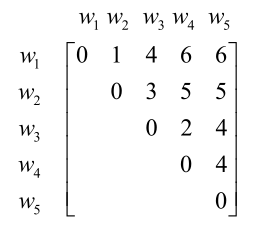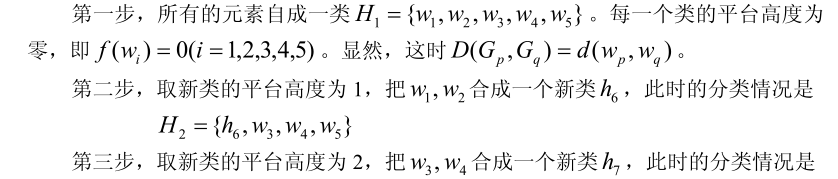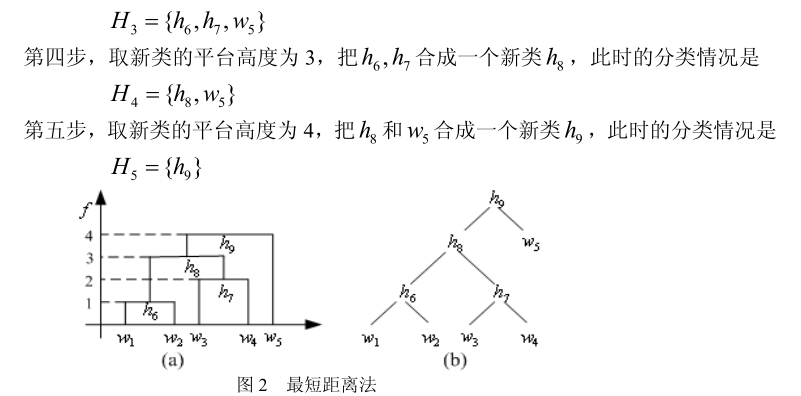# matlab代码详解

clc,clear
a=[1,0;1,1;3,2;4,3;2,5];
[m,n]=size(a);
d=zeros(m);
for i=1:m
for j=i+1:m
d(i,j)=mandist(a(i,:),a(j,:)');
%求第一个矩阵的行向量与第二个矩阵的列向量之间对应的绝对值距离
end
end
d
nd=nonzeros(d); %去掉d中的零元素，非零元素按列排列
nd=union(nd,nd) %去掉重复的非零元素
for i=1:m-1
nd_min=min(nd);
[row,col]=find(d==nd_min);tm=union(row,col); %row和col归为一类
tm=reshape(tm,1,length(tm)); %把数组tm变成行向量
fprintf('第%d次合成，平台高度为%d时的分类结果为：%s\n',...
i,nd_min,int2str(tm));
nd(find(nd==nd_min))=[]; %删除已经归类的元素
if length(nd)==0
break
end
end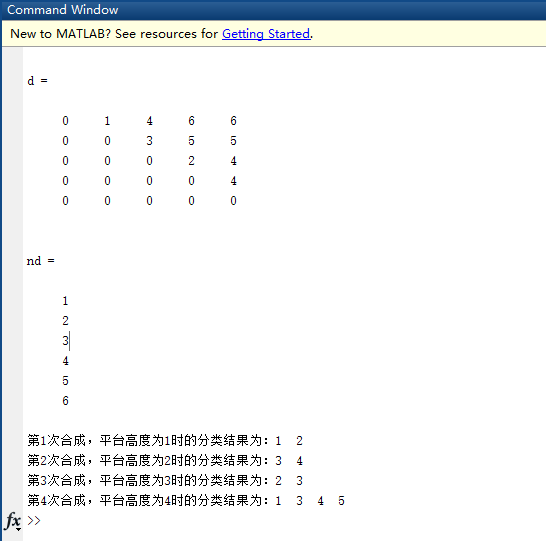clc,clear
a=[1,0;1,1;3,2;4,3;2,5];
y=pdist(a,'cityblock'); %求a的两两行向量间的绝对值距离
yc=squareform(y) %变换成距离方阵
z=linkage(y) %产生等级聚类树
[h,t]=dendrogram(z) %画聚类图
T=cluster(z,'maxclust',3) %把对象划分成3类
for i=1:3
tm=find(T==i); %求第i类的对象
tm=reshape(tm,1,length(tm)); %变成行向量
fprintf('第%d类的有%s\n',i,int2str(tm)); %显示分类结果
end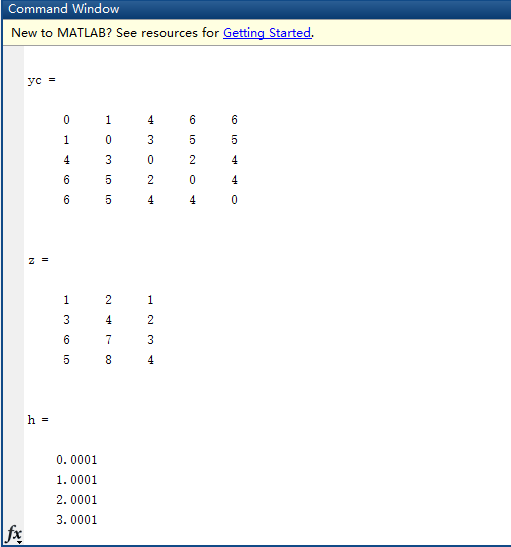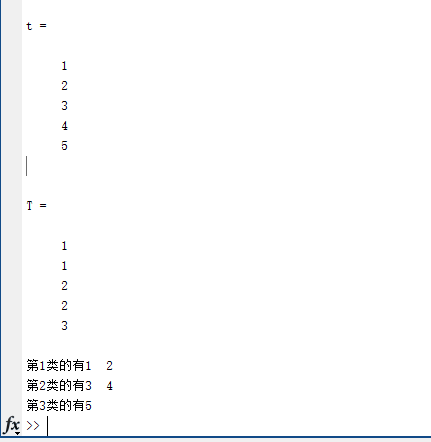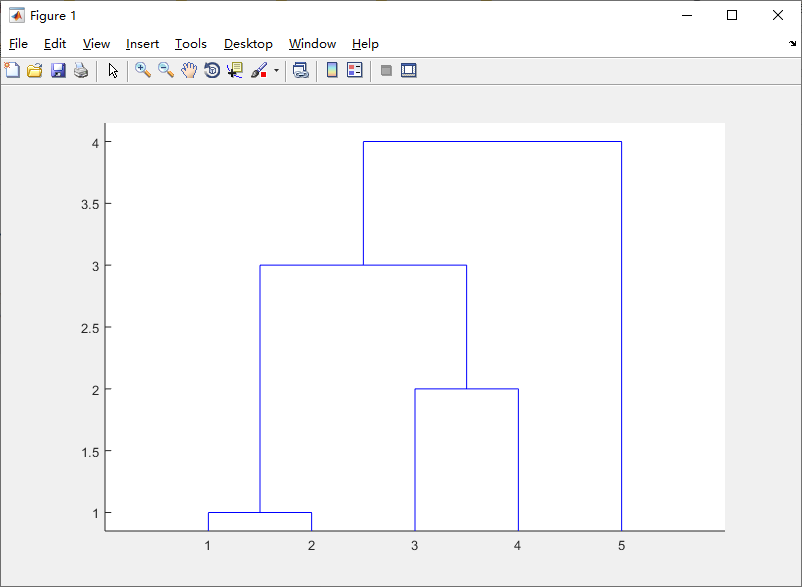转载规则

《数据分析模型（二）：模糊聚类分析方法及实例（附完整代码）》 梦魇回生 采用 知识共享署名 4.0 国际许可协议 进行许可。
上一篇Matlab串联矩阵函数【horzcat】详细解析

2020-12-07教你在python中用不同的方式画不同颜色的画布

2020-12-05
目录